# Exploring IBM HR data using Python

## Intro

This tutorial series explores the IBM HR data set. This data is typically used to demonstrate the ability of various machine learning algorithms applied to HR data.

In this series, I'll use it to demonstrate the awesome power Python can bring to HR data

Sections

• Statistics
• Matplotlib
• Pandas
• Seaborn
• Plotly
• Findings
In :
__author__ = "adam"
__version__ = "1.0.0"

In :
# imports
import numpy as np
import pandas as pd

import matplotlib.pyplot as plt

%matplotlib inline

In :
# if continuing on from the previous section, read the data from saved file


In :
# read the data directly from IBM Watson Analytics
# using pandas read excel file into dataframe

# save data for later
# empl_data.to_excel("WA_Fn-UseC_-HR-Employee-Attrition.xlsx")


### pandas

Pandas? We've already looked at pandas. Yes, but we've not explored the plotting capabilities of pandas.

This section, let's explore Gender.

In :
empl_data['Gender'].value_counts(normalize=True).plot(kind='bar', title='Employee Gender')

Out:
<matplotlib.axes._subplots.AxesSubplot at 0x26e9a422d30>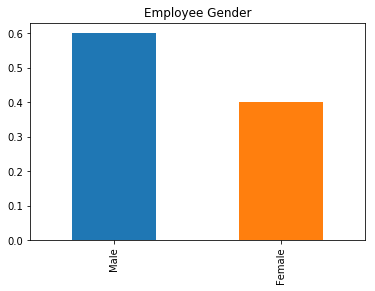~60% of the employees are Male, ~40% Female.

#### Salary</mh4>

In :
empl_data.pivot_table(values='HourlyRate', columns='Gender', aggfunc='mean')\
.plot(kind='bar', title='Average Hourly Salary by Gender and Job Level')

Out:
<matplotlib.axes._subplots.AxesSubplot at 0x26e9a593c88>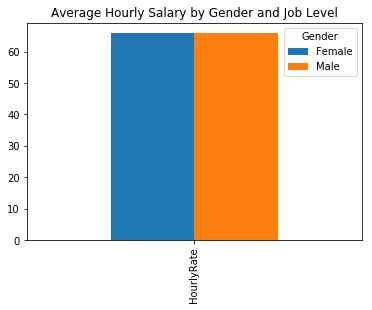Positively, there does not appear to be any variance in the overall average pay between gender.

Let's explore further

In :
# by job level
empl_data.pivot_table(values='HourlyRate',index='JobLevel', columns='Gender', aggfunc='mean')\
.plot(kind='bar', title='Average Hourly Salary by Gender and Job Level')

Out:
<matplotlib.axes._subplots.AxesSubplot at 0x26e9a858668>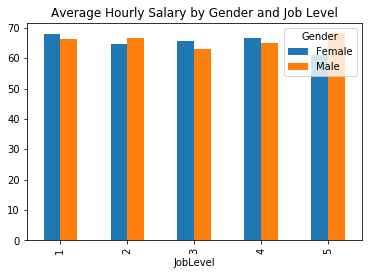Again, no significant findings. Let's look at one more...

In :
# by department
empl_data.pivot_table(values='HourlyRate',index='Department', columns='Gender', aggfunc='mean')\
.plot(kind='bar', title='Average Hourly Salary by Gender and Department')

Out:
<matplotlib.axes._subplots.AxesSubplot at 0x26e9a8bb470>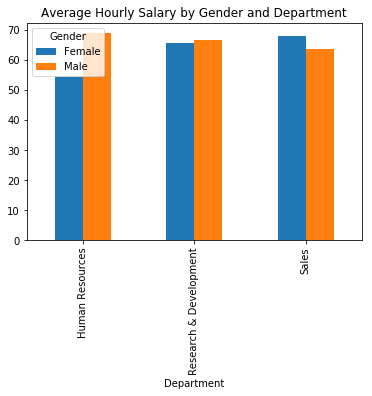Here, it would initially appear that within Sales there is a a significant pay gap for Female employees.

#### Performance

In :
empl_data.pivot_table(values='PerformanceRating', columns='Gender', aggfunc='mean')\
.plot(kind='bar', title='Average Performance Rating by Gender and Job Level')

Out:
<matplotlib.axes._subplots.AxesSubplot at 0x26e9b90fe48>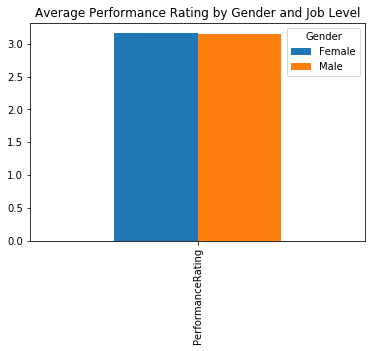Performance also appears equal between Genders. What about by Job Level?

In :
empl_data.pivot_table(values='PerformanceRating',index='JobLevel', columns='Gender', aggfunc='mean')\
.plot(kind='bar', title='Average Performance Rating by Gender and Job Level')

Out:
<matplotlib.axes._subplots.AxesSubplot at 0x26e9a3db978>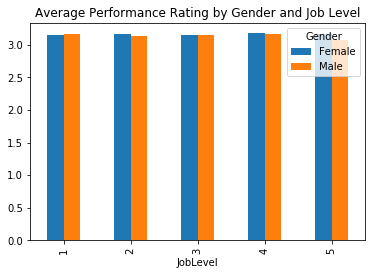And now, what about by Department? Will we see the same as with Average Salary?

In :
empl_data.pivot_table(values='PerformanceRating',index='Department', columns='Gender', aggfunc='mean')\
.plot(kind='bar', title='Average Performance Rating by Gender and Department')

Out:
<matplotlib.axes._subplots.AxesSubplot at 0x26e9a3daf98>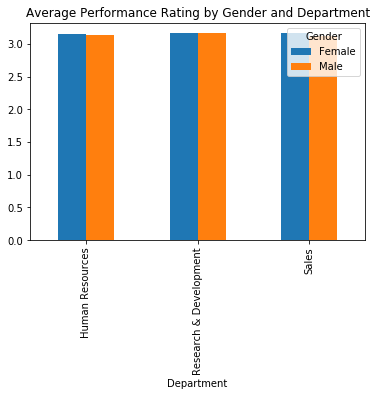Average Performance appears equal - even in Sales; this does not explain the salary difference between genders.

In :
empl_data.columns

Out:
Index(['Age', 'Attrition', 'BusinessTravel', 'DailyRate', 'Department',
'DistanceFromHome', 'Education', 'EducationField', 'EmployeeCount',
'EmployeeNumber', 'EnvironmentSatisfaction', 'Gender', 'HourlyRate',
'JobInvolvement', 'JobLevel', 'JobRole', 'JobSatisfaction',
'MaritalStatus', 'MonthlyIncome', 'MonthlyRate', 'NumCompaniesWorked',
'Over18', 'OverTime', 'PercentSalaryHike', 'PerformanceRating',
'RelationshipSatisfaction', 'StandardHours', 'StockOptionLevel',
'TotalWorkingYears', 'TrainingTimesLastYear', 'WorkLifeBalance',
'YearsWithCurrManager'],
dtype='object')
In :
empl_data.pivot_table(values='HourlyRate',index='PerformanceRating', columns='Gender', aggfunc='mean')\
.plot.barh(title="Average Performance Rating by Gender")

Out:
<matplotlib.axes._subplots.AxesSubplot at 0x26e9a321550>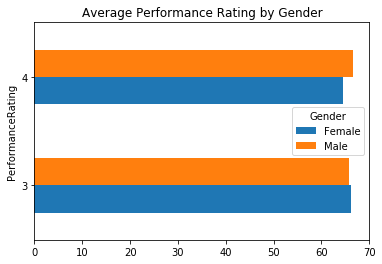With pandas, we can even strip away some of the transformations and call methods directly on the DataFrame.

In :
empl_data.boxplot(column='HourlyRate', by='Gender', grid=False)

Out:
<matplotlib.axes._subplots.AxesSubplot at 0x26e9b95ad30>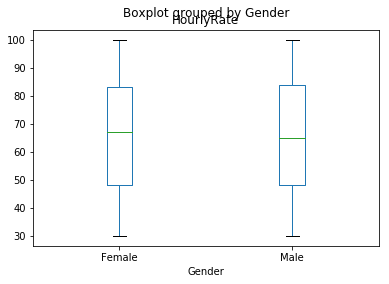In :
# suppress warning message from matplotlib
import warnings; warnings.simplefilter('ignore')


In :
empl_data[empl_data['Gender'] == 'Male']['HourlyRate'].plot.hist(by='Gender', alpha=0.5, normed=True)
empl_data[empl_data['Gender'] == 'Female']['HourlyRate'].plot.hist(by='Gender', alpha=0.5, normed=True)

Out:
<matplotlib.axes._subplots.AxesSubplot at 0x26e9b99fd68>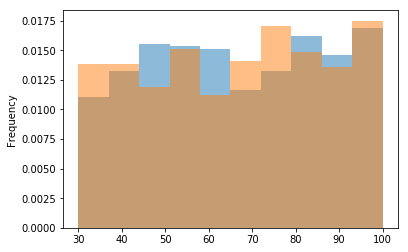### Section Findings

Having explored Education, we learned:

• Compensation does not vary between genders significantly.
• Compensation variance is not noted across Job Level nor Department.
• Performance Ratings generally appear similar across gender.

### Pandas Recap

Pandas plotting is powerful; you have the ability to plot directly from your DataFrames.

Here's the secret - pandas doesn't do any plotting. As was mentioned in the previous section, matplotlib, other packages build on top of matplotlib. pandas is no exception. In fact, the .plot method is just a wrapper around matplotlib calls.

Still, this can be more effective that calling matplotlib directly. When working with DataFrames, it's easy to transform the data and pass to .plot matplotlib via the wrapper methods.